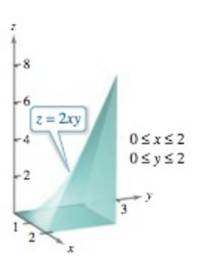Chapter 14.6, Problem 20E

Chapter
Section
Textbook Problem

Volume In Exercises 19-24, use a triple integral to find the volume of the solid bounded by the graphs of the equations.To determine

To calculate: The volume of the solid bounded by with the help of Triple integral if the graphs of the equations z=2xy;x=0;x=2;y=0;y=2 and z=0

Explanation

Given:

The given 3-D plot shown below;

Formula used:

The integration formula is,

abxndx=[xn+1n+1]ab

Calculation:

Consider the provided 3-D plot,

From the provided 3-D plot, z varies from 0 to 2xy, x varies from 0 to 2 and y varies from 0 to 2. Thus, the volume of the solid can be calculated by this expression 020202xydzdxdy as below,

020202xyd</

Still sussing out bartleby?

Check out a sample textbook solution.

See a sample solution

The Solution to Your Study Problems

Bartleby provides explanations to thousands of textbook problems written by our experts, many with advanced degrees!

Get Started

Simplify the expressions in Exercises 97106. 32/331/6

Finite Mathematics and Applied Calculus (MindTap Course List)

In Exercises 1124, find the indicated limits, if they exist. 23. limx3x2+2x+42x23x+1

Applied Calculus for the Managerial, Life, and Social Sciences: A Brief Approach

Using n = 4 and midpoints for xi, then Riemann sum for 17x2 dx is a) 3443 b) 72 c) 168 d) 112

Study Guide for Stewart's Single Variable Calculus: Early Transcendentals, 8th

The distance from (1, 2, 1) to the plane 6x + 5y + 8z = 34 is:

Study Guide for Stewart's Multivariable Calculus, 8th

Graph: 3x+2y6.

College Algebra (MindTap Course List)